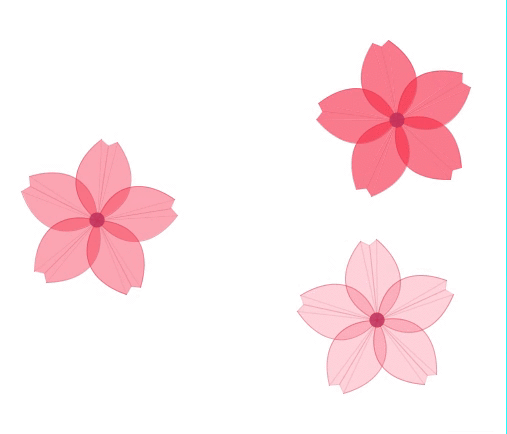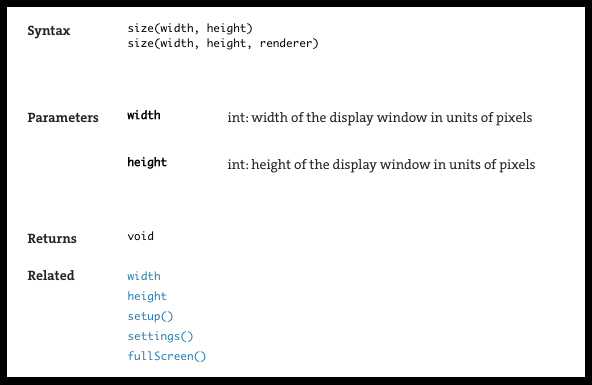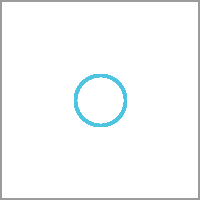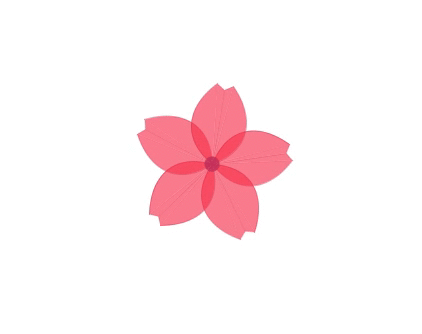# [Processing × Java] How to use the function1. Pre-prepared functions
2. Functions that you define and create
3. Example of function modularization → reuse

# 0. What is a function?

## 0-0. ** What is a function **

A function is like an output device. It takes something, converts it and outputs it.

## 0-1. ** Why use functions **

The main reason is ① ** Because the program becomes easier to read. ** ** ② ** Because it can be reused. ** ** (Details on reuse later)

## 0-2. ** Function type **

There are two types of functions: "** pre-prepared functions " and " self-defined functions **".

The functions provided in advance are, specifically, size (), background (), line (), void setup (), void draw () ... etc.

# 1. ** Pre-prepared functions **

In Processing, ** many functions are prepared in advance **. size (), background (), rect (), ellipse () ... etc. For example, in the size () function, size (600,400); will display a window with a width of 600px and a height of 400px.

The important thing here is that the pre-made functions must be used according to ** the rules **.

For example, size (600,400,300,200); will result in an error. You can find the ** set rules ** by looking at the Processing Reference.

(Example) Fixed rule of size ()# 2. ** Functions that you define and create **

Programming allows you to create your own original functions to simplify your program. First, we will simplify the program that displays the following circles.

#### `maru.java`

``````

void setup(){
size(200,200);
background(255);
}

void draw(){
strokeWeight(3);
stroke(77,196,224);
noFill();
ellipse(100,100,30,30);
}
``````To simplify the program ① ** Modularize ** ② ** Make it reusable ** I will do that.

## ① Modularize

#### `maru_module.java`

``````

void setup(){
size(200,200);
background(255);
}

//You can see at a glance what to repeat!
void draw(){
//Execute the original function you created.
maru();
}

//Summarized here! !!
void maru(){
strokeWeight(3);
stroke(77,196,224);
noFill();
ellipse(100,100,30,30);
}
``````

Point : By combining ** into one function **, the draw () function will be cleaner and the program will be easier to understand.

## ② Make it reusable

Reusable means that ** multiple outputs are possible **.

#### `maru_reuse.java`

``````
void setup(){
size(200,200);
background(255);
}

void draw(){
//You can easily display multiple circles!
//maru(x coordinate,y coordinate,The size of the circle);
maru(100,80,100);
maru(100,100,50);
}

//Function definition
//First decide what to take as an argument
void maru(float x,float y,float s){
strokeWeight(3);
stroke(77,196,224);
noFill();
//It says what to use the argument value for
ellipse(x,y,s,s);
}

``````Point : To make it reusable, allow the value of the argument (parameter) to be determined. That way, you can change the output value of the function.

for that purpose (1) Decide which value you want to change and express it as a variable.

``````  ellipse(x,y,s,s);
``````

(2) Define the argument in the form of ** data type variable **.

``````void maru(float x,float y,float s){
}
``````

③ Use the function according to the rules of the function you defined.

``````  maru(100,80,100);
``````

### □ ** What is void **

The data received as the result of executing the function is called ** return value **.

Functions basically return values such as int and float. Example of a function that returns a float type number ↓

#### `float_function.java`

``````
void setup(){
//Self-defined average()Use a function to assign the mean value to the variable f.
float f = average(12.0,6.0);
//Display the value of f.
println(f);
}

//A function that can find the average of two values average()To define.
float average(float num1,float num2){
//Create an expression so that the mean value of two values can be assigned to the variable av.
float av = (num1 + num2) / 2.0;
//Returns a float type number as the return value
return av;
}
``````
``````9.0
``````

However, functions that draw shapes, such as ellipse (), do not return a value. Use void in this way when the function ** does not return a return value **.

Point : Functions that do not return a return value are usually used by themselves. (That is, void is not written) background (), fill (), rect () .... etc. Add void to the function ** that determines the processing content using ** {}. void setup () {}, void draw () {} ... etc.

# 3. Example of function modularization → reuse

### ◯ Program to draw cherry blossom petals

#### `sakura.java`

``````
int x = 300;
int y = 300;
//Define a variable r that determines the speed of rotation.
float r = 30.0;
//Define the variable a that determines the transparency of the cherry blossoms.
int a = 150;

void setup(){
size(600,600);
}

void draw(){
background(255);
//The depictions up to popMatrix are output together.
pushMatrix();
//Coordinate reference point(origin)To(x,y)To move to
translate(x,y);
//Use the variable r to determine how much to rotate.
rotate(frameCount/r);
//Depiction of cherry blossoms
for(int i = 0;i < 5;i++){
rotate(TWO_PI * 1/5);
noStroke();
fill(255,50,80,a);
stroke(225,50,80,100);
bezier(0,0,35,-20,35,-52,8,-80);
bezier(0,0,-35,-20,-35,-52,-8,-80);
stroke(225,50,80,30);
triangle(0,0,8,-80,0,-75);
triangle(0,0,-8,-80,0,-75);
}
fill(200,50,100,200);
ellipse(0,0,15,15);
//The depiction of cherry blossoms is output together.
popMatrix();
}
``````### ◯ Program to draw cherry blossom petals (modularize)

#### `sakura_module.java`

``````
int x = 300;
int y = 300;
float r = 30.0;
int a = 150;

void setup(){
size(600,600);
}

//draw()The inside of the function is cleaner and easier to see.
void draw(){
background(255);
sakura();
}

//Define a function for cherry blossom petals.
void sakura(){
pushMatrix();
translate(x,y);
rotate(frameCount/r);
for(int i = 0;i < 5;i++){
rotate(TWO_PI * 1/5);
noStroke();
fill(255,50,80,a);
stroke(225,50,80,100);
bezier(0,0,35,-20,35,-52,8,-80);
bezier(0,0,-35,-20,-35,-52,-8,-80);
stroke(225,50,80,30);
triangle(0,0,8,-80,0,-75);
triangle(0,0,-8,-80,0,-75);
}
fill(200,50,100,200);
ellipse(0,0,15,15);
popMatrix();
}

``````

### ◯ Program to draw cherry blossom petals (make it reusable)

#### `sakura_reuse.java`

``````
void setup(){
size(600,600);
}

void draw(){
background(255);
//Output 3 functions with different parameters
sakura(400,200,30,150);
sakura(100,300,-40,100);
sakura(380,400,60,50);
}
//Define the original function
//Arguments are defined in the form of data types and variables.
void sakura(float x,float y,float r,float a){

pushMatrix();
translate(x,y);
rotate(frameCount/r);
for(int i = 0;i < 5;i++){
rotate(TWO_PI * 1/5);
noStroke();
fill(255,50,80,a);
stroke(225,50,80,100);
bezier(0,0,35,-20,35,-52,8,-80);
bezier(0,0,-35,-20,-35,-52,-8,-80);
stroke(225,50,80,30);
triangle(0,0,8,-80,0,-75);
triangle(0,0,-8,-80,0,-75);
}
fill(200,50,100,200);
ellipse(0,0,15,15);
popMatrix();
}
``````# Finally

Thank you for reading. We appreciate your opinions and suggestions in order to make the article even better.